## 12.1 The ast module

The ast module contains specialist functions for astronomy and cosmology.

ast.Lcdm_­age(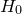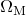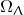)
The ast.Lcdm_­age() function returns the current age of the Universe in a standard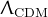cosmology with specified values for Hubble’s constant,and. Hubble’s constant should be specified either with physical units of recession velocity per unit distance, or as a dimensionless number, assumed to have implicit units of km/s/Mpc. Suitable input values for a standard cosmology are: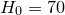,and. For more details, see David W. Hogg’s short article Distance measures in cosmology, available online at:
http://arxiv.org/abs/astro-ph/9905116.

ast.Lcdm_­angscale()
The ast.Lcdm_­angscale() function returns the angular scale of the sky at a redshift ofin a standardcosmology. For details, see the ast.Lcdm_age() function above. The returned value has dimensions of distance per unit angle.

ast.Lcdm_­DA()
The ast.Lcdm_­DA() function returns the angular size distance of objects at a redshift ofin a standardcosmology. For details, see the ast.Lcdm_age() function above. The returned value has dimensions of distance.

ast.Lcdm_­DL()
The ast.Lcdm_­DL() function returns the luminosity distance of objects at a redshift ofin a standardcosmology. For details, see the ast.Lcdm_age() function above. The returned value has dimensions of distance.

ast.Lcdm_­DM()
The ast.Lcdm_­DM() function returns the proper motion distance of objects at a redshift ofin a standardcosmology. For details, see the ast.Lcdm_age() function above. The returned value has dimensions of distance.

ast.Lcdm_­t()
The ast.Lcdm_­t() function returns the lookback time to objects at a redshift ofin a standardcosmology. For details, see the ast.Lcdm_age() function above. The returned value has dimensions of time. To find the age of the Universe at a redshift of, this value should be subtracted from the output of the ast.Lcdm_age() function.

ast.Lcdm_­z()
The ast.Lcdm_­z() function returns the redshift corresponding to a lookback time ofin a standardcosmology. For details, see the ast.Lcdm_age() function above. The returned value is dimensionless.

ast.moonphase()
The ast.moonphase() function returns the phase of the Moon, with dimensions of angle, at the time of the supplied date object. Ifis numerical, it is assumed to be a Unix time.

ast.sidereal_­time()
The ast.sidereal_­time() function returns the sidereal time at Greenwich, with dimensions of angle, at the time of the supplied date object. Ifis numerical, it is assumed to be a Unix time. The returned sidereal time is equal to the right ascension of the stars which are transiting the Greenwich meridian at that time. This function uses the expression for sidereal time adopted in 1982 by the International Astronomical Union (IAU), and which is reproduced in Chapter 12 of Jean Meeus’ book Astronomical Algorithms (1998).